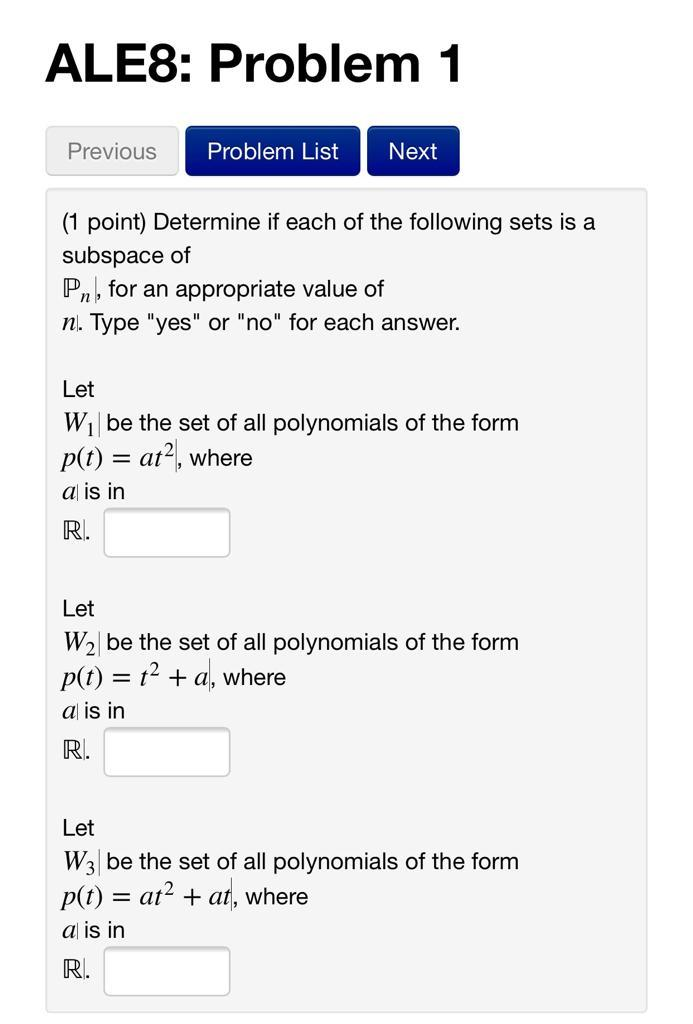### Create an Account

Already have account?

### Forgot Your Password ?

Home / Questions / ALES: Problem 1 Previous Problem List Next (1 point) Determine if each of the following se...

# ALES: Problem 1 Previous Problem List Next (1 point) Determine if each of the following sets is a subspace of Pn, for an appropriate value of n. Type "yes" or "no" for each answer. Let W be the set of

ALES: Problem 1 Previous Problem List Next (1 point) Determine if each of the following sets is a subspace of Pn, for an appropriate value of n. Type "yes" or "no" for each answer. Let W be the set of all polynomials of the form p(t) = ata, where a is in R. Let W2 be the set of all polynomials of the form p(t) = 12 + a, where a is in R. Let W3 be the set of all polynomials of the form p(t) = ata + at, where a is in R.Apr 15 2021 View more View Less

#### Answer (Solved)Subscribe To Get Solution